# Consistent And Inconsistent Linear System

Some linear systems have no solution; they are called inconsistent systems. If a linear system has at least one solution than it is called consistent system.

To illustrate this with example, consider two linear equations that represents two line on xy-plane given below.

\begin{aligned}
&a_{1}x_{1} + a_{2}x_{2} = b_{1}\\
&a_{3}x_{1} + a_{4}x_{2} = b_{2}
\end{aligned}

where either one variable can be zero, not both.

Let us call the lines as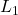and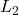. A solution to the equation is a point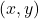that satisfy both equations given above.

### Possibilities of Solution

There are three possibilities of solution for lines –and.

• No Solutions
• Exactly One Solution
• Infinite Solutions

Let us examine each one of these possibilities closely.

#### No Solutions

If the lines –andare parallel, that is, not touching each other, there is no possibility of a common point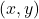.

Therefore, the above system has no solution.

#### Exactly One Solution

Suppose the lines –andintersect each other exactly at a point. Then we have exactly one solution to the linear system.

The pointin the above diagram is the only solution that satisfy equation of both the lines.

#### Infinite Solutions

When the two lines –andare the same or overlap each other then we can say that there could be more than one pointsbelong to both line.

Therefore, the equation has infinite solutions because the line may increase forever in the xy-plane. We can formally say that ” Every linear system has no solution, one solution, or infinitely many solutions“.

### Augmented Matrix

We know linear equations and system of linear equations from our earlier discussions. Any system of linear equation with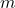equations and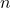variable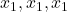, …,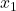is written as

\begin{aligned}
&a_{11}x_{1} + a_{12}x_{2}+ a_{13}x_{3} = b_{1}\\
&a_{21}x_{1} + a_{22}x_{2}+ a_{23}x_{3} = b_{2}\\
&a_{31}x_{1} + a_{32}x_{2}+ a_{33}x_{3} = b_{3}
\end{aligned}

where a’s and b’s are real number constants.

The same equations can be represented using a two-dimensional system called augmented matrix. We usually refer as ‘matrix‘,but the word ‘augmented’ highlights that context of matrix system. We now know from where the matrix came from.

\begin{bmatrix}a_{11} & a_{12} & ... & a_{1n} & b_{1}\\ a_{21} & a_{22} & ... & a_{2n} & b_{2}\\: & : & : & : & :\\a_{m1} & a_{m2} & ... & a_{mn} & b_{m}\end{bmatrix}

The double subscript indicate the correct position of a term with respect to rows and columns where rows are equations and columns are terms.

Important points

Here are some important points to consider while constructing a augmented matrix form a system of equations.

• keep the order of a’s according to unknown variables x’s.
• keep the b constant on the right in the same order as in the equation.

Example

Problem #1 : Change the following system of linear equations to augmented matrix.

\begin{aligned}
&3x_{1} + x_{2}+ 6x_{3} = 1\\
&2x_{1} + 2x_{2}+ x_{3} = 7\\
&4x_{1} - 6x_{2}+ 9x_{3} = 5
\end{aligned}

Solution : The resultant augmented matrix is

\begin{bmatrix}3 & 1 & 6 & 1\\ 2 & 2 & 1 & 7\\ 4 & -6 & 9 & 5\end{bmatrix}

We will consider changing the terms with the help of basic row operations in the next post.